CUET  >  Test: Problems On Ages - 2

# Test: Problems On Ages - 2 - CUET

Test Description

## 20 Questions MCQ Test General Test Preparation for CUET - Test: Problems On Ages - 2

Test: Problems On Ages - 2 for CUET 2023 is part of General Test Preparation for CUET preparation. The Test: Problems On Ages - 2 questions and answers have been prepared according to the CUET exam syllabus.The Test: Problems On Ages - 2 MCQs are made for CUET 2023 Exam. Find important definitions, questions, notes, meanings, examples, exercises, MCQs and online tests for Test: Problems On Ages - 2 below.
Solutions of Test: Problems On Ages - 2 questions in English are available as part of our General Test Preparation for CUET for CUET & Test: Problems On Ages - 2 solutions in Hindi for General Test Preparation for CUET course. Download more important topics, notes, lectures and mock test series for CUET Exam by signing up for free. Attempt Test: Problems On Ages - 2 | 20 questions in 40 minutes | Mock test for CUET preparation | Free important questions MCQ to study General Test Preparation for CUET for CUET Exam | Download free PDF with solutions
 1 Crore+ students have signed up on EduRev. Have you?
Test: Problems On Ages - 2 - Question 1

### The sum of the present ages of a son and his father is 60 years. Six years ago, father's age was five times the age of the son. After 6 years, what will be son's age?

Detailed Solution for Test: Problems On Ages - 2 - Question 1

Let the present age of the son = x Then, present age of the father = ( 60 − x ) Six years ago father's age was 5 times the age of the son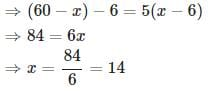Son's age after 6 years = x + 6 = 14 + 6 = 20

Test: Problems On Ages - 2 - Question 2

### Kiran is younger than Bineesh by 7 years and their ages are in the respective ratio of 7 : 9. How old is Kiran?

Detailed Solution for Test: Problems On Ages - 2 - Question 2

Let age of Kiran and Bineesh be 7x and  9x  respectively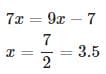Kiran's age = 7 x = 7 × 3.5 = 24.5

Test: Problems On Ages - 2 - Question 3

### Six years ago, the ratio of the ages of Vimal and Saroj was 6 : 5 . Four years hence, the ratio of their ages will be 11 : 10 . What is Saroj's age at present?

Detailed Solution for Test: Problems On Ages - 2 - Question 3

Given that, six years ago, the ratio of the ages of Vimal and Saroj = 6 : 5
Hence we can assume that age of Vimal six years ago = 6x
age of Saroj six years ago = 5x
After 4 years, the ratio of their ages = 11 : 10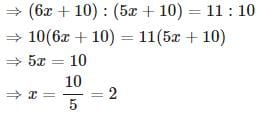Saroj's present age

= ( 5x + 6 ) = 5 x 2 + 6 = 16

Test: Problems On Ages - 2 - Question 4

At present, the ratio between the ages of Shekhar and Shobha is 4 : 3 . After 6 years, Shekhar's age will be 26 years. Find out the age of Shobha at present?

Detailed Solution for Test: Problems On Ages - 2 - Question 4

After 6 years, Shekhar's age will be 26 years
Therefore, Present age of Shekhar = 26 − 6 = 20
Let present age of Shobha = x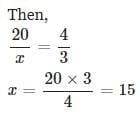Test: Problems On Ages - 2 - Question 5

My brother is 3 years elder to me. My father was 28 years of age when my sister was born while my mother was 26 years of age when I was born. If my sister was 4 years of age when my brother was born, then what was the age of my father when my brother was born?

Detailed Solution for Test: Problems On Ages - 2 - Question 5

Let my age = x
Then
My brother's age = x + 3
My mother's age = x + 26
My sister's age = ( x + 3 ) + 4 = x + 7 My father's age = ( x + 7 ) + 28 = x + 35
Age of my father when my brother was born = x + 35 − ( x + 3 ) = 32

Test: Problems On Ages - 2 - Question 6

The present ages of A,B and C are in proportions 4 : 7 : 9 . Eight years ago, the sum of their ages was 56 . What are their present ages (in years)?

Detailed Solution for Test: Problems On Ages - 2 - Question 6

Let present age of A,B and C be 4 x , 7 x and 9 x respectively.
(4x−8)+(7x−8)+(9x−8)=56
⇒20x=80
⇒x=4
Hence present age of A, B and C are
4 × 4 , 7 × 4 and 9 × 4 respectively.
i.e., 16 , 28 and 36 respectively.

Test: Problems On Ages - 2 - Question 7

A person's present age is two-fifth of the age of his mother. After 8 years, he will be one-half of the age of his mother. What is the present age of the mother?

Detailed Solution for Test: Problems On Ages - 2 - Question 7

Let present age of the person =x
Then, present age of the mother = 5x/2
Given that, after  8  years, the person will be one-half of the age of his mother.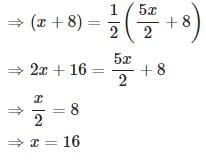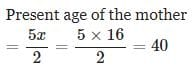Test: Problems On Ages - 2 - Question 8

A is as much younger than B and he is older than C. If the sum of the ages of B and C is 50 years, what is definitely the difference between B and A's age?

Detailed Solution for Test: Problems On Ages - 2 - Question 8

Age of C < Age of A < Age of B

Given that sum of the ages of B and C is 50 years.

Now we need to find out (B's age - A's age). But this cannot be determined with the given data.

Test: Problems On Ages - 2 - Question 9

Sobha's father was 38 years of age when she was born while her mother was 36 years old when her brother four years younger to her was born. What is the difference between the ages of her parents?

Detailed Solution for Test: Problems On Ages - 2 - Question 9

Let Sobha's age = x and
her brother's age = x − 4
Sobha's father's age = x + 38
Sobha's mother's age = ( x − 4 ) + 36 = x + 32
Sobha's father's age - Sobha's mother's age
=(x+38)−(x+32)=6

Test: Problems On Ages - 2 - Question 10

The age of father 10 years ago was thrice the age of his son. Ten years hence, father's age will be twice that of his son. What is the ratio of their present ages?

Detailed Solution for Test: Problems On Ages - 2 - Question 10

Let age of the son before 10 years = x and  age of the father before 10 years = 3 x
(3x + 20 ) = 2 ( x + 20 )
⇒ x = 20
Age of the son at present = x + 10 = 20 + 10 = 30
Age of the father at present = 3 x + 10 = 3 × 20 + 10 = 70
Required ratio = 70 : 30 = 7 : 3

Test: Problems On Ages - 2 - Question 11

The ages of two persons differ by 16 years. 6 years ago, the elder one was 3 times as old as the younger one. What are their present ages of the elder person?

Detailed Solution for Test: Problems On Ages - 2 - Question 11

Let present age of the elder person = x and
present age of the younger person = x − 16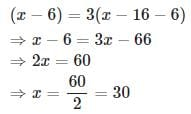Test: Problems On Ages - 2 - Question 12

Present age of a father is 3 years more than three times the age of his son. Three years hence, father's age will be 10 years more than twice the age of the son. What is father's present age?

Detailed Solution for Test: Problems On Ages - 2 - Question 12

Let the present age the son = x

Then, present age of the father = 3 x + 3

Given that, three years hence, father's age will be 10 years more than twice the age of the son

⇒ ( 3 x + 3 + 3 ) = 2 ( x + 3 ) + 10

⇒ x = 10

Father's present age

= 3 x + 3 = 3 × 10 + 3 = 33

Test: Problems On Ages - 2 - Question 13

Kamal was 4 times as old as his son 8 years ago. After 8 years, Kamal will be twice as old as his son. Find out the present age of Kamal.

Detailed Solution for Test: Problems On Ages - 2 - Question 13

Let age of the son before 8 years = x

Then, age of Kamal before 8 years ago = 4x

After 8 years, Kamal will be twice as old as his son

⇒ 4 x + 16 = 2 ( x + 16 )

⇒ x = 8

Present age of Kamal = 4 x + 8 = 4 × 8 + 8 = 40

Test: Problems On Ages - 2 - Question 14

If 6 years are subtracted from the present age of Ajay and the remainder is divided by 18 , then the present age of Rahul is obtained. If Rahul is 2 years younger to Denis whose age is 5 years, then what is Ajay's present age?

Detailed Solution for Test: Problems On Ages - 2 - Question 14

Present age of Denis = 5 years

Present age of Rahul = 5 − 2 = 3

Let present age of Ajay = x

Then, present age of Rahul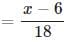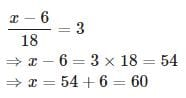Test: Problems On Ages - 2 - Question 15

The ratio of the age of a man and his wife is 4 : 3 . At the time of marriage the ratio was 5 : 3 and After 4 years this ratio will become 9 : 7 . How many years ago were they married?

Detailed Solution for Test: Problems On Ages - 2 - Question 15

Let the present age of the man and his wife be 4 x and 3 x respectively.

After 4 years this ratio will become 9 : 7 ⇒ ( 4 x + 4 ) : ( 3 x + 4 ) = 9 : 7
⇒ 7 ( 4 x + 4 ) = 9 ( 3 x + 4 )
⇒ 28 x + 28 = 27 x + 36
⇒ x = 8

Present age of the man = 4 x = 4 × 8 = 32
Present age of his wife = 3 x = 3 × 8 = 24
Assume that they got married before t years. Then,
( 32 − t ) : ( 24 − t ) = 5 : 3
⇒ 3 ( 32 − t ) = 5 ( 24 − t )
⇒ 96 − 3 t = 120 − 5 t
⇒ 2 t = 24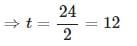Test: Problems On Ages - 2 - Question 16

The product of the ages of Syam and Sunil is 240 . If twice the age of Sunil is more than Syam's age by 4 years, what is Sunil's age?

Detailed Solution for Test: Problems On Ages - 2 - Question 16

Let age of Sunil = x
and age of Syam = y
xy = 240 ⋯ ( 1 )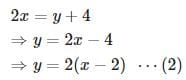Substituting equation ( 2 ) in equation ( 1 ) . We get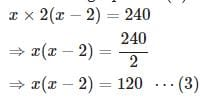We got a quadratic equation to solve.
Always time is precious and objective tests measure not only how accurate you are but also how fast you are. We can solve this quadratic equation in the traditional way. But it is more easy to substitute the values given in the choices in the quadratic equation (equation 3 ) and see which choice satisfy the equation.
Here, option A is 10 . If we substitute that value in the quadratic equation, x ( x − 2 ) = 10 × 8 which is not equal to 120
Now try option B which is 12 . If we substitute that value in the quadratic equation, x ( x − 2 ) = 12 × 10 = 120 . See, we got that x = 12
Hence Sunil's age = 12
(Or else, we can solve the quadratic equation by factorization as,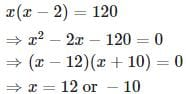Since x is age and cannot be negative, x = 12
Or by using quadratic formula as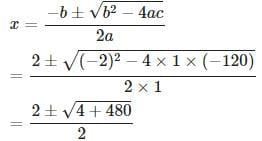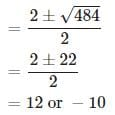Since age is positive, x = 12

Test: Problems On Ages - 2 - Question 17

One year ago, the ratio of Sooraj's and Vimal's age was 6 : 7 respectively. Four years hence, this ratio would become 7 : 8 . How old is Vimal?

Detailed Solution for Test: Problems On Ages - 2 - Question 17

Let the age of Sooraj and Vimal, 1 year ago, be 6 x and 7 x respectively.
Given that, four years hence, this ratio would become 7 : 8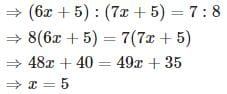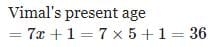Test: Problems On Ages - 2 - Question 18

The total age of A and B is 12 years more than the total age of B and C. C is how many year younger than A?

Detailed Solution for Test: Problems On Ages - 2 - Question 18

Given that, A + B = 12 + B + C

⇒ A - C = 12

Therefore, C is younger than A by 12 years

Test: Problems On Ages - 2 - Question 19

Sachin's age after 15 years will be 5 times his age 5 years back. Find out the present age of Sachin?

Detailed Solution for Test: Problems On Ages - 2 - Question 19

Let present age of Sachin = x . Then,
( x + 15 ) = 5 ( x − 5 )
⇒ 4 x = 40
⇒ x = 10

Test: Problems On Ages - 2 - Question 20

Sandeep's age after six years will be three-seventh of his father's age. Ten years ago the ratio of their ages was 1 : 5 . What is Sandeep's father's age at present?

Detailed Solution for Test: Problems On Ages - 2 - Question 20

Let the age of Sandeep and his father before 10 years be x and 5 x respectively.
Given that Sandeep's age after six years will be three-seventh of his father's age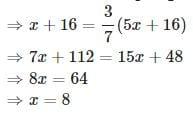Sandeep's father's present age
= 5 x + 10 = 5 × 8 + 10 = 50

## General Test Preparation for CUET

187 videos|404 docs|867 tests
Information about Test: Problems On Ages - 2 Page
In this test you can find the Exam questions for Test: Problems On Ages - 2 solved & explained in the simplest way possible. Besides giving Questions and answers for Test: Problems On Ages - 2, EduRev gives you an ample number of Online tests for practice

## General Test Preparation for CUET

187 videos|404 docs|867 tests(Scan QR code)